# tencent cloud

### Using Kubecost for TKE Cost Management

Last updated: 2021-08-18 11:01:01

## Overview

As the middle layer of IaaS and PaaS, the billing of Kubernetes currently relies on the resource billing of IaaS. A Kubernetes cluster is billed by the purchased node CVMs. However, users use the Pods. In actual scenarios, users are often faced with questions such as how does a Pod bear the cost, how to evaluate the cost of the cluster, how to make cost predictions and optimization suggestions. For the above problems, we recommend users to use Kubecost.

Kubecost is a cost analysis tool that can provide insights, analysis, recommendations and suggestions on cluster costs. As your financial analyst for optimizing cluster costs, Kubecost can provide you with a comprehensive cost analysis report. This document describes the Kubecost use cases, optimization suggestions, detailed features, and how to install and use it.

## Use Cases

### Evaluating the consumption cost of each resource

The cost expenditure is the cost of calculating Pod resource request (Request) or usage (Usage). The cost of Pod is calculated based on the IaaS billing method of the node where the Pod is located.

Currently, the node billing modes of cloud vendors are generally monthly subscription (Month), pay-as-you-go (Hour) and spot instance. When you use Kubecost to calculate the cost of Pod, even containers with the same requests have different costs on different types of nodes.

In the above three billing modes, user purchases an entire instance. The billing is based on the instance, not for a single resource. By using Kubecost, you can refer to the model to analyze the apportioned cost of each resource type and each resource.

For example, a cloud vendor provides a node (virtual machine or physical machine) with 1 CPU core (C), 1 GB memory (Mem), and a price of 20 CNY/month.

By using Kubecost, you need to add the basic price of each resource specified by the cloud vendor, such as the CPU and Mem prices, or configure the corresponding ratio based on the business needs, for example, the price ratio of 1 Core:1 GB is 3:1, CPU/Mem=3:1. You can know the billing allocated to each resource (CPU/GPU/Mem/PV/Network).

The calculation formula is as follows:
sum (normalized_resource_price[i] × resource_quantity[i]) = node_price

Therefore, the price of the entire node is 20 CNY/month, and the apportioned cost is 15 CNY/month for CPU and 5 CNY/month for Mem.

### Evaluating cost efficiency

You can evaluate the cost efficiency via cost weighted average. Because the cost weight of each resource is different (cost weight means that different types of resources are sold at different prices. For example, the price of computing resources such as CPU and GPU is relatively high, while the price of Mem is relatively low, and the price of disk is even lower), the contribution of different resources to the cost is also different for the same resource utilization efficiency.

For example, if the disk utilization is 100%, since the disk is relatively cheap, the contribution to the final cost control is low. However, for the CPU resources, even if the resource utilization rate is 30%, since the price of CPU resources is relatively expensive, it may play a key role in the cost. Therefore, it is necessary to use weighted average to evaluate the cost efficiency, for example:

• Mem efficiency: MemEfficiency = MemUsage/MemRequest
• CPU efficiency: CPUEfficiency = cpuUsage / cpuRequest
• Mem cost efficiency: MemCostEff = a.MemEfficiency() × a.MemTotalCost()
• CPU cost efficiency: cpuCostEff = a.CPUEfficiency() × a.CPUTotalCost()
• Total cost efficiency: totalEff = (MemCostEff + cpuCostEff) / (a.CPUTotalCost() + a.MemTotalCost())

### Optimization suggestions

When judging which services need to be optimized and how to optimize the cost structure, you can first look for the TOP 10 resource wastes. For example, the Usage of resources is very different from the Request. You can obtain the recommended Request based on the application monitoring profile, and finally calculate each resource costs that can be saved.

### Evaluating the marginal cost

For the node auto scaling, you can measure the final cost if the CPU and Mem of each node in the cluster increase by 1 core and 1 GB, and whether there will be a scale effect (the scale effect means that when the number of resources increases, the cost does not explode, but the bin packing problem is solved).

If you have the above requirements and problems, you can use Kubecost to analyze your cluster cost trends.

## Prerequisites

• You have created a TKE cluster. For how to create a cluster, see Creating a Cluster.
• You have used the command line tool Kubectl to connect to the cluster. For how to connect to a cluster, see Connecting to a Cluster.

## Features

• Kubecost mainly provides cost analysis, including label dimension analysis such as Service, Application, Pod, and Workload.
• Resource allocation and usage
• It provides a cluster health check feature, which is similar to cluster inspection and health check.

### Overview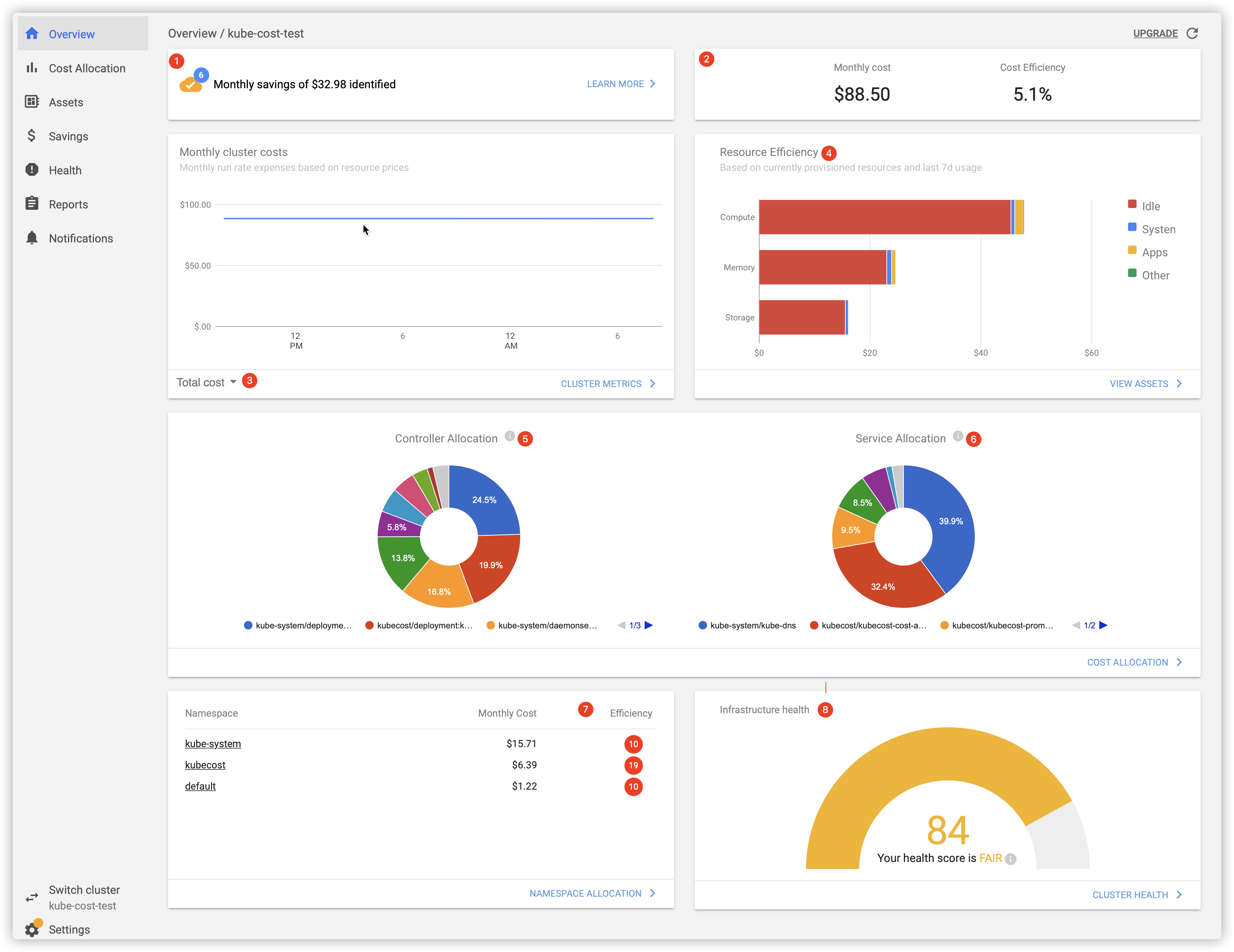The corresponding description for each part is as follows:

1. The estimated monthly savings and the number of optimization suggestions for cost savings
2. Monthly cost and cost efficiency:
• Monthly cost: the predicted value based on resource consumption over the past 7 days.
• Cost efficiency: the cost efficiency based on last 2 days.
Calculation formula: (utilization rate of each resource * the sum of each resource’s price) / total prices
3. Monthly cluster costs. The bill dimensions include “Total cost”, “Compute”, “Memory”, and “Storage”.
4. Resource efficiency:
• Based on currently provisioned resources and last 7 days usage
• The resources are divided into three dimensions: Compute, Memory, and Storage.
• Cost consists of four dimensions: idle, system, apps, and others.
5. Controller Allocation: calculate the cost of each Controller in the last two days based on the classification of Controllers.
6. Service Allocation: calculate the cost of each Service in the last two days based on the classification of Services.
7. Namespace Allocation: calculate the cost and cost efficiency of each namespace in the last two days based on the classification of namespace.
Calculation formula: utilization of each resource utilization * the sum of each resource’s price / total prices
8. Infrastructure health: the cluster infrastructure status score, which is similar to the cluster inspection, and there are some optimization suggestions, for example:
• Worker nodes are deployed across AZs.
• Master has multiple replicas.
• Detect the Pod whose CPU is throttled.

### Cost allocation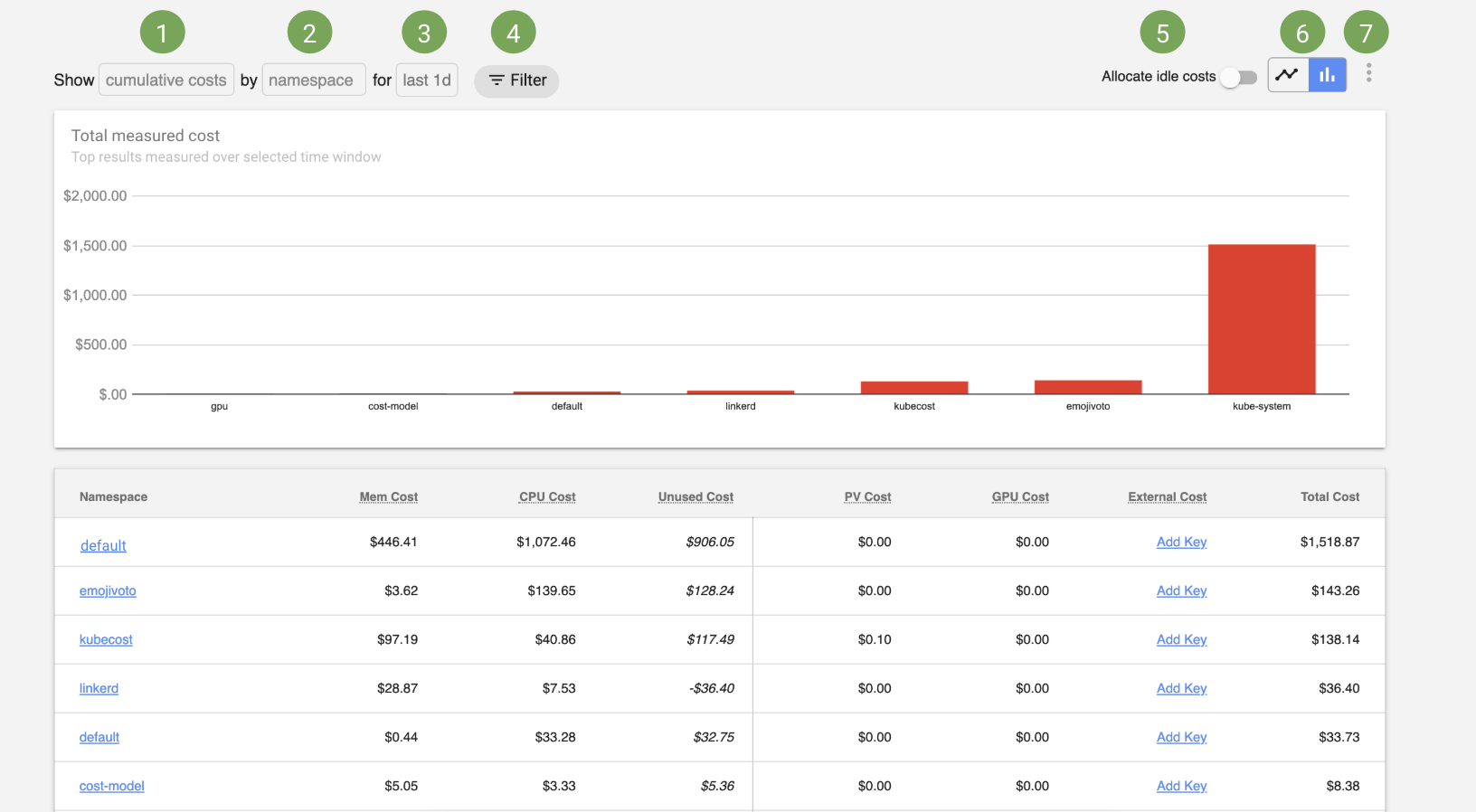The corresponding description for each part is as follows:

1. Displayed metrics:
• Cumulative costs: the actual or historical expenditures over the selected time window.
• Rate metrics: the hourly, daily, or monthly cost, which is based on samples in the selected time window, and used to estimate costs.
2. Aggregation:
Cost Allocation allows you to view the allocation expenditure all native Kubernetes concepts, such as NS, Label, and Service. It also supports the cost allocation for the organizational concepts such as team, Product/project, Department, or environment.
3. Time window:
The specified time window used to measure costs. By default, the queried results of 1 day, 2 days, 7 days, and 30 days are cached.
4. Filter:
Filter resources by NS, clusterId, label, and Pod Prefix to accurately query the key points of cost expenditure.
5. Allocate idle costs:
“Allocate idle costs” will allocate idle resources and idle cluster costs to tenants in proportion, which is applicable to the resources that are configured but not fully used or requested by the tenant. For example, if your cluster is only 25% utilized, measured by the maximum usage of resources and Requests, “Allocate idle costs” will proportionally increase the cost of each pod/NS/Deployment to 4 times.
6. Selecting charts:
You can switch to the bar chart to view the total measured cost of the selected window, or switch to the sequence diagram to view the cost changes over time.
Export the cost data into CSV files or view the help documentations.

### Assets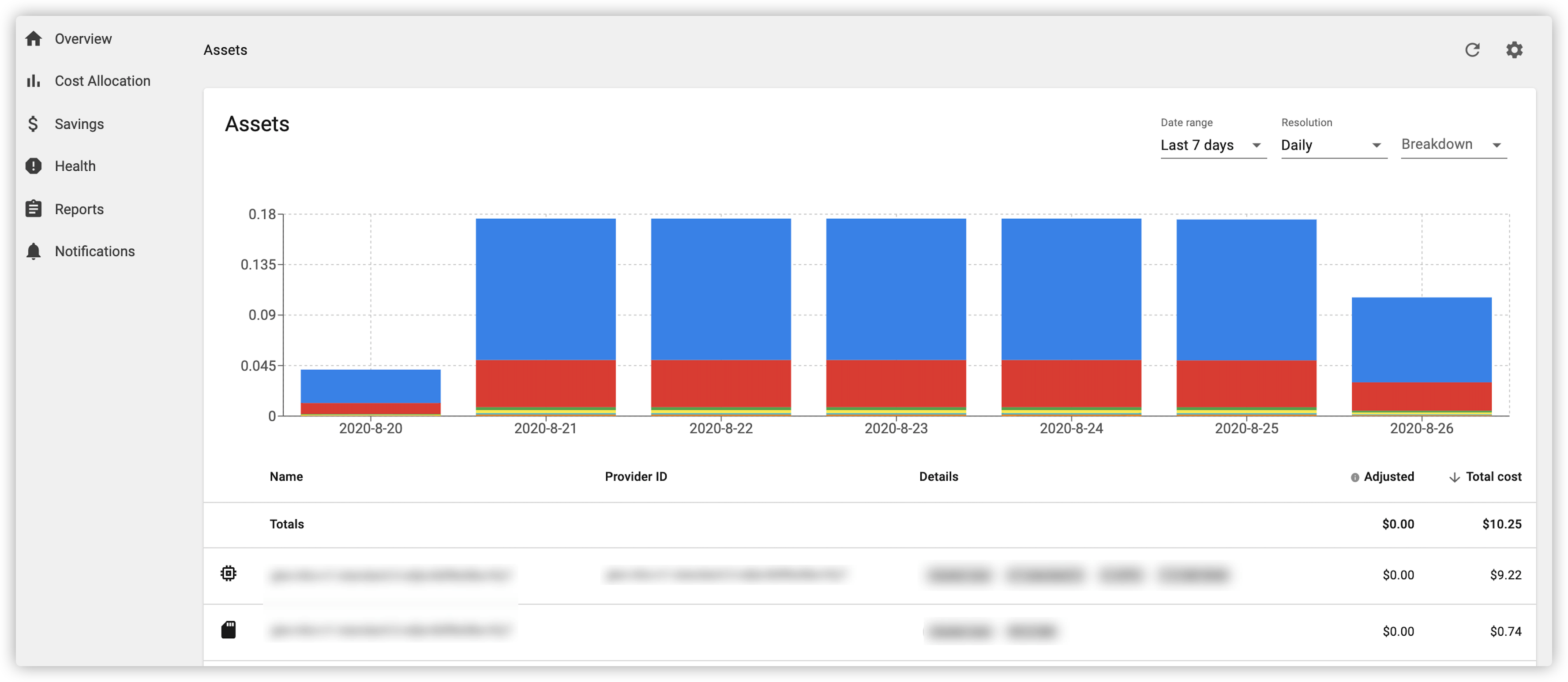The Kubecost Assets view shows the fine-grained cost allocation of a Kubernetes cluster based on a single resource in the cluster (for example, the cost allocated by nodes, disks, and other resources).

### Savings

It shows the monthly estimated savings and the number of optimization suggestions for cost savings, namely the first entry in the “Overview” page.

Here takes Request Sizing Recommendations as an example, as shown in the second figure below. The following three levels of recommendation values are provided, and the recommended values are also related to the given time window:

• *Development: *the aim is 80% resource utilization at 85th-percentile resource usage over the given window.
• *Production: *the aim is 65% resource utilization at 98th-percentile resource usage over the given window.
• *High-availability: *the aim is 50% resource utilization at 99.9th-percentile resource usage over the given window.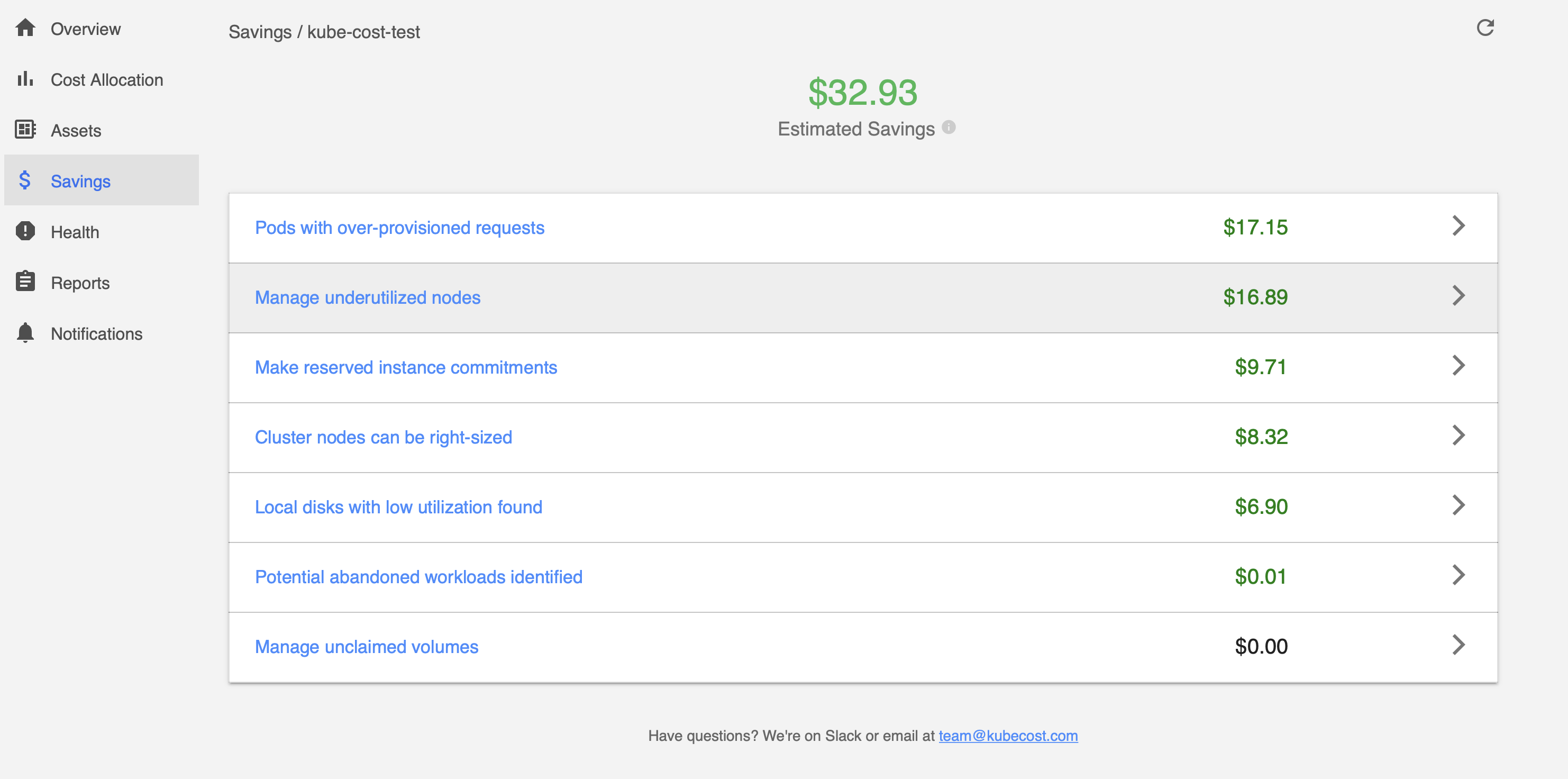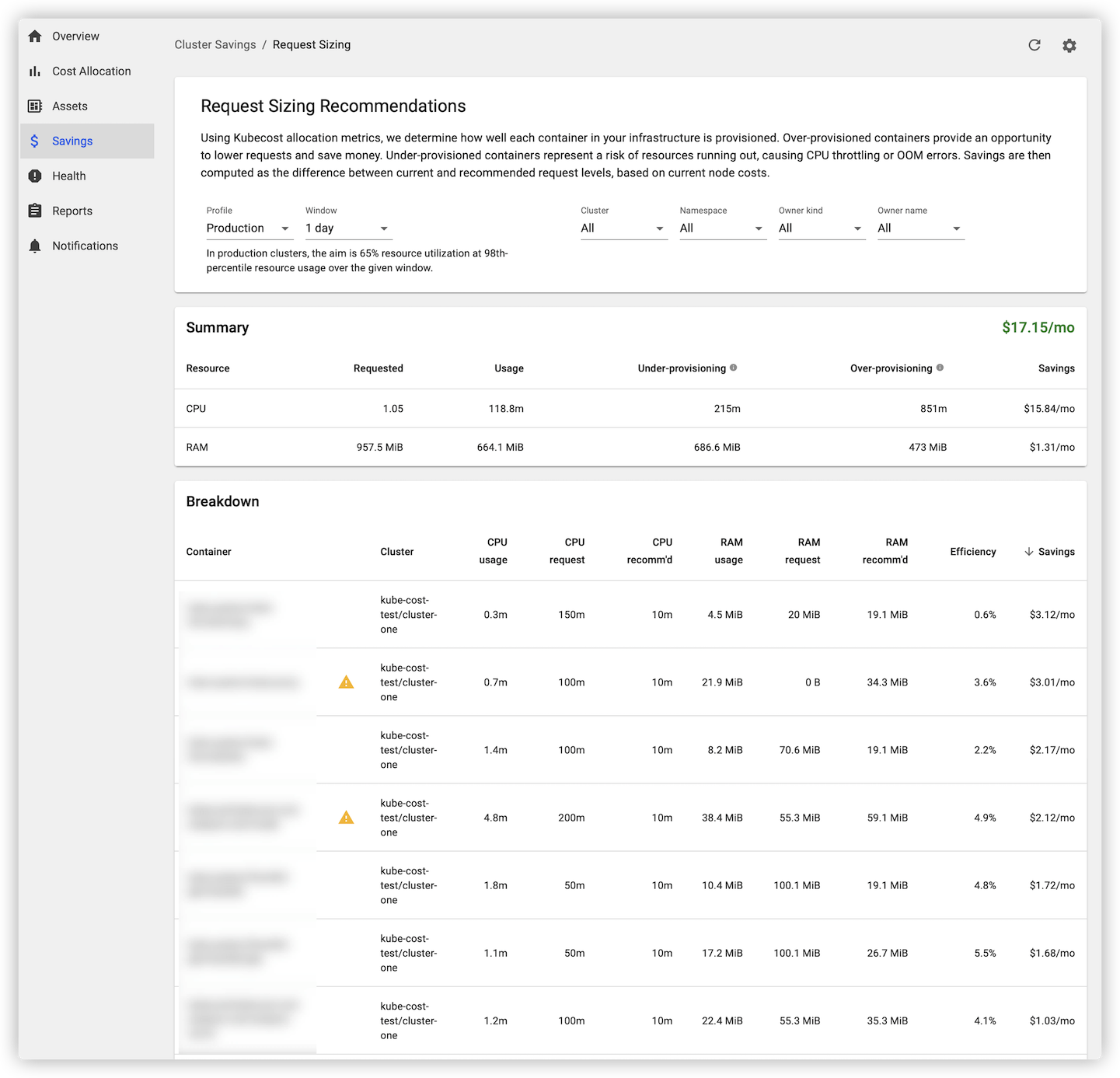### Health

The score of the cluster infrastructure status. If there are suggested repairs, it will be marked with a red exclamation point ❗, as shown in the figure below: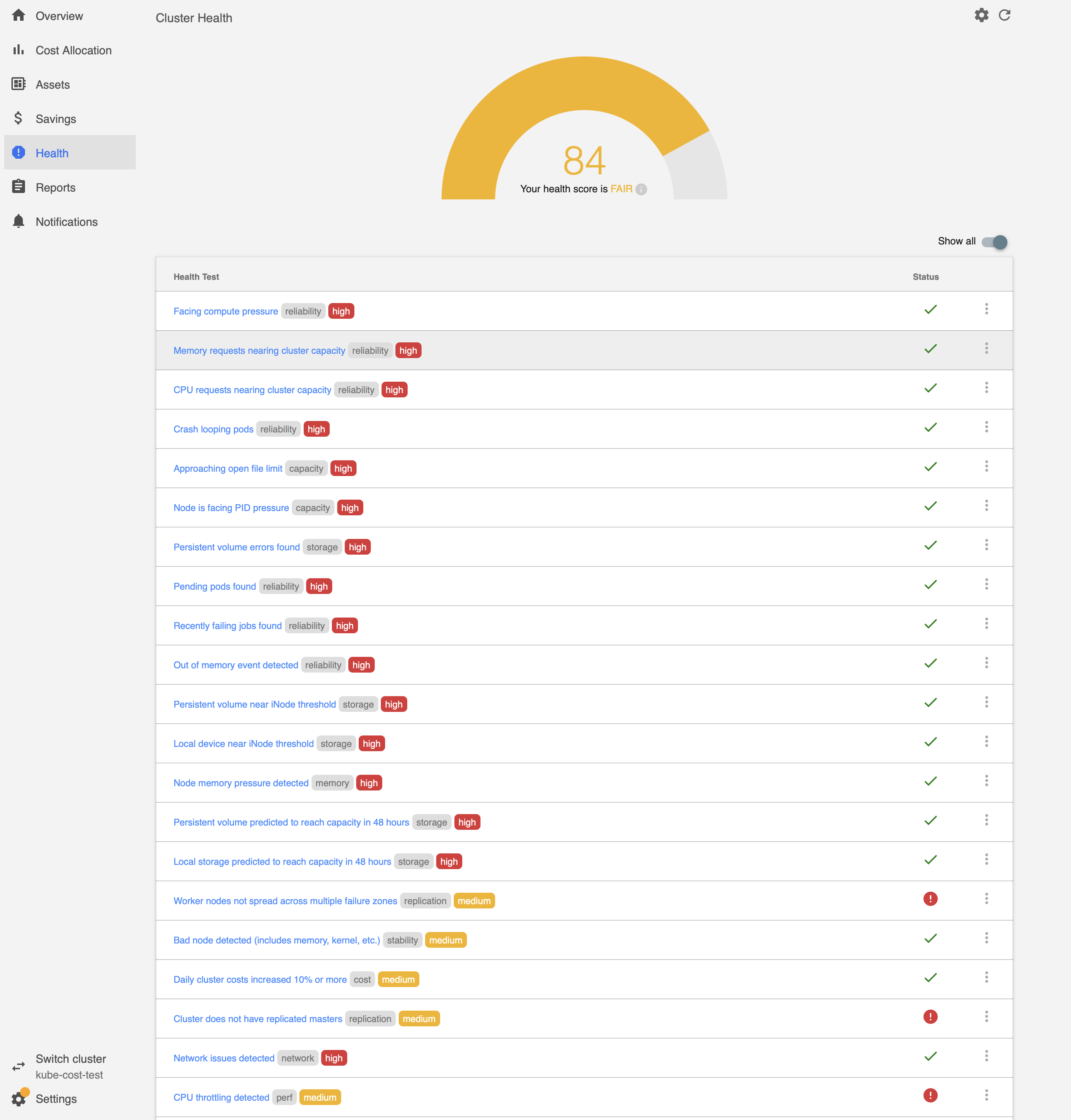### Reports

Reports are mainly used to save the observation data. The observation granularity is the same as that in Cost Allocation. It supports data storage with one-click after data aggregation/observation in a certain way, as shown below: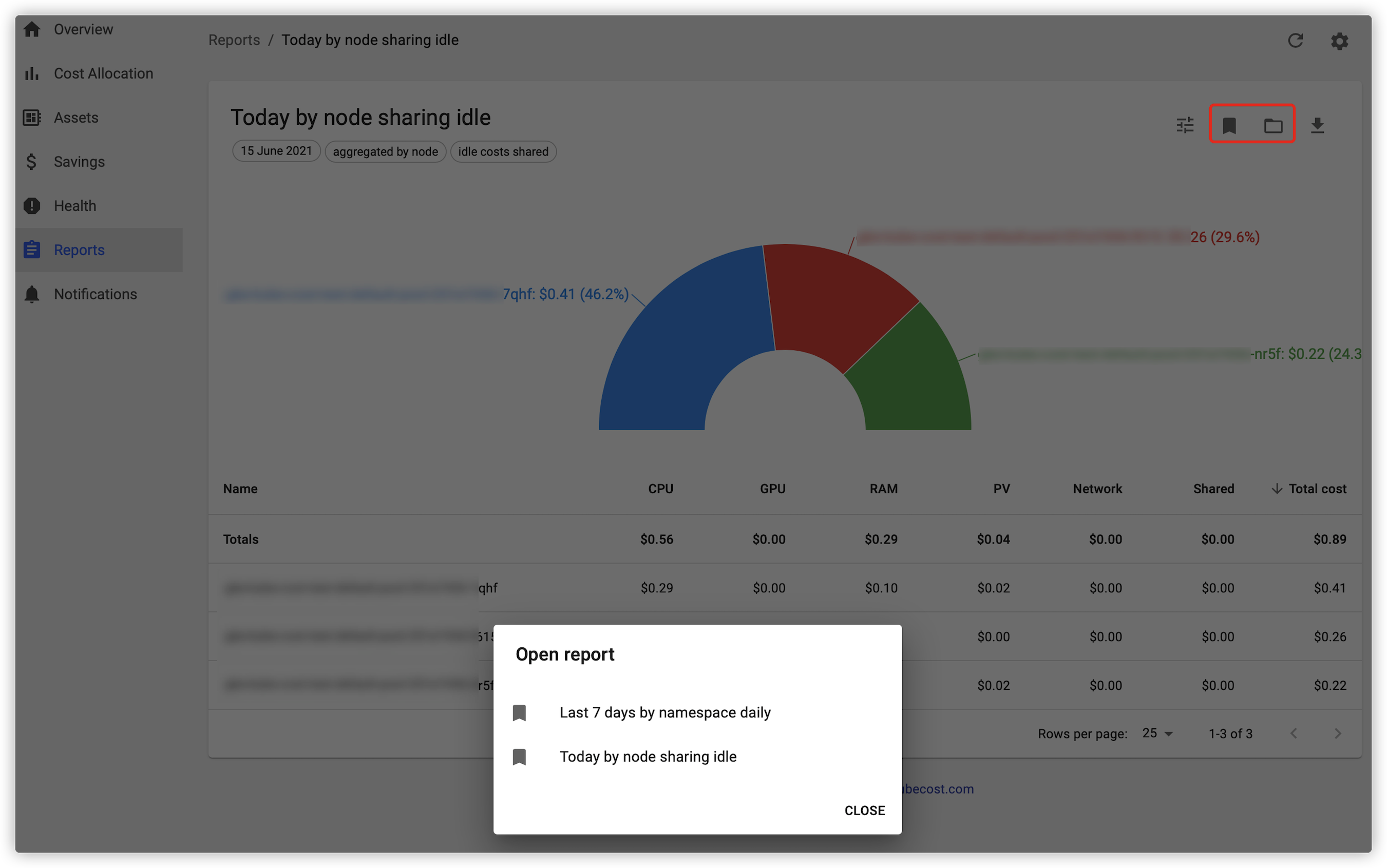## Directions

### Installing Helm

Log in to a node and run the following command to install Helm.

``````curl https:``//raw.githubusercontent.com/helm/helm/master/scripts/get-helm-3 | bash
``````

``````wget https:``//qitian-1251707795.cos.ap-beijing.myqcloud.com/cost-analyzer-1.81.0.tgz
``````

### Installing Kubecost

1. Run the following command to install Kubecost:

``````kubectl create ns kubecost``helm install cost-analyzer cost-analyzer-``1.81``.``0``.tgz -n kubecost
``````
2. Run the following command to check whether the Pods are running properly, as shown below:

``````kubectl get pods -n kubecost -o wide
``````

The execution result is as shown below: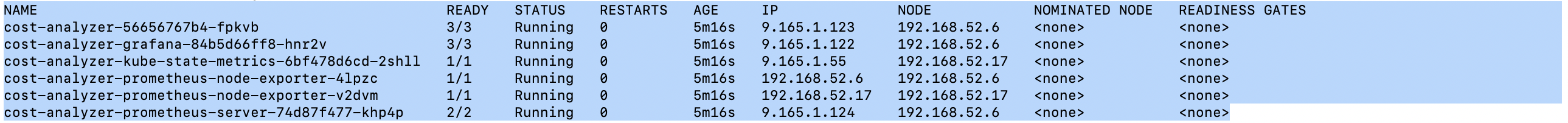### Updating service access method

2. Click the corresponding cluster ID/name to go to the “Cluster Management” page.
3. Select Services and Routes > Service to go to the “Service” page.
4. Select the Service that you want to update the access method, and click Update access method to go to the “Update access method” page.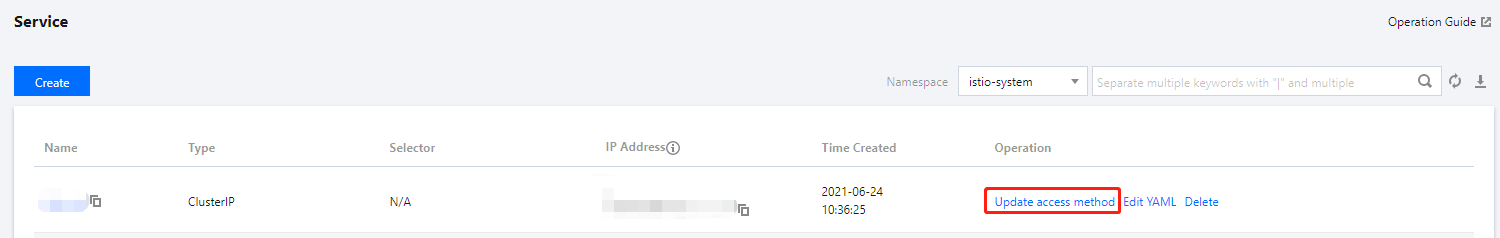5. The access method of service cost-analyzer-cost-analyzer is load balancer. After the service access method is updated, you can obtain a public IPv4 address for public network access.

### Uninstalling

Run the following command to uninstall Kubecost:

``````helm uninstall cost-analyzer -n kubecost
``````